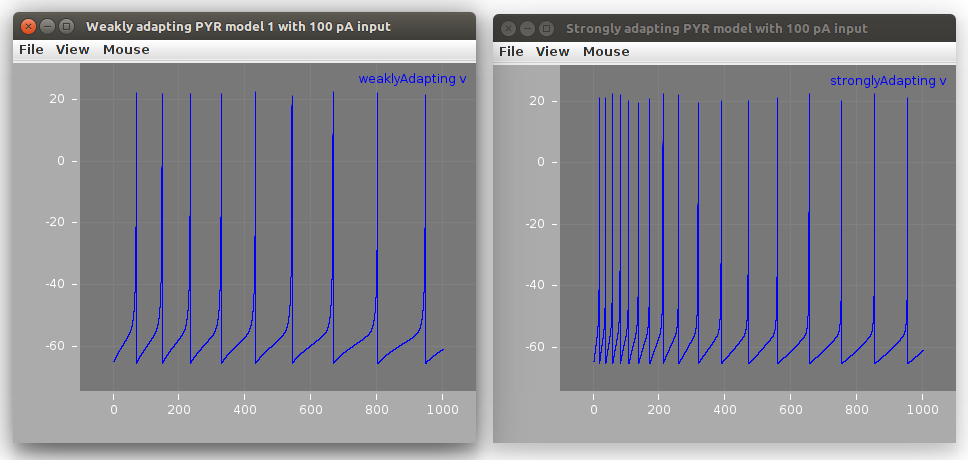CA1 pyramidal cell - Ferguson et al. 2014
 Contributors

## CA1 pyramidal cell - Ferguson et al. 2014¶

Ferguson KA, Huh CY, Amilhon B, Williams S, Skinner FK (2014) Simple, biologically-constrained CA1 pyramidal cell models using an intact, whole hippocampus context. F1000Res 3:104

Izhikevich-based models of CA1 pyramidal cells, with parameters constrained based on a whole hippocampus preparation. Strongly and weakly adapting models based on the experimental data have been developed. Code produces example model output.

### NeuroML2/LEMS version of model¶

The model defined in the Python Brian scripts has been converted to a LEMS ComponentType defintion: IzhikevichFerguson.xml.

Note this is different from the existing Izhikevich model definition in NeuroML2, as outlined in Ferguson et al. 2014:

The model has a fast variable representing the membrane potential, V (mV),
and a slow “recovery” current given by the variable u (pA).
In order to capture the spike width at threshold, we slightly modified the
Izhikevich model by using a different “k” parameter above
and below the spike threshold (khigh and klow respectively).

A LEMS file (LEMS_TwoCells.xml) creates a network with 2 instances of the cells:

```<izhikevichFergusonCell id="weaklyAdapting" v0 = "-65mV" C="300 pF" vr = "-61.8 mV"
vpeak = "22.6 mV" c = "-65.8 mV" klow = "0.5 nS_per_mV" khigh = "3.3 nS_per_mV"
a = "0.001 per_ms" d = "5 pA" vt = "-57.0 mV" b = "3 nS"/>

<izhikevichFergusonCell id="stronglyAdapting" v0 = "-65mV" C="115 pF" vr = "-61.8 mV"
vpeak = "22.6 mV" c = "-65.8 mV" klow = "0.1 nS_per_mV" khigh = "3.3 nS_per_mV"
a = "0.0012 per_ms" d = "10 pA" vt = "-57.0 mV" b = "3 nS"/>
```

applies currents as specified in the Python scripts and runs a simulation with the cells:This model can be run locally using jNeuroML:

```git clone https://github.com/OpenSourceBrain/FergusonEtAl2014-CA1PyrCell.git # Clone GitHub repository
cd FergusonEtAl2014-CA1PyrCell/NeuroML2
jnml LEMS_TwoCells.xml
```

or using pyNeuroML:

```git clone https://github.com/OpenSourceBrain/FergusonEtAl2014-CA1PyrCell.git # Clone GitHub repository
cd FergusonEtAl2014-CA1PyrCell/NeuroML2
pynml LEMS_TwoCells.xml
```